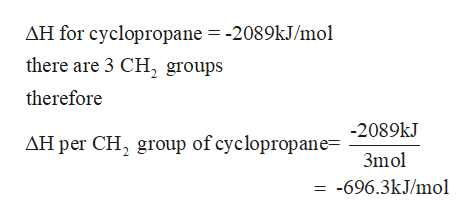# The molar heat of combustion of gaseous cyclopropane is -2089 Kj/mol, that for gaseous cyclopentene is -3317 Kj/mol. Calculate the heat of combustion per CH2 group in the two cases and account for the difference

Question
4 views

The molar heat of combustion of gaseous cyclopropane is -2089 Kj/mol, that for gaseous cyclopentene is -3317 Kj/mol. Calculate the heat of combustion per CH2 group in the two cases and account for the difference

check_circle

Step 1

The structure of cyclopropane is

Step 2

There are three CH2 groups in the molecule of cyclopropane. Molar heat of combustion per CH2 group ishelp_outlineImage TranscriptioncloseAH for cyclopropane = -2089kJ/mol there are 3 CH, groups therefore -2089kJ AH per CH, group of cyclopropane= 3mol = -696.3kJ/mol fullscreen
Step 3

The structure of cyc...

### Want to see the full answer?

See Solution

#### Want to see this answer and more?

Solutions are written by subject experts who are available 24/7. Questions are typically answered within 1 hour.*

See Solution
*Response times may vary by subject and question.
Tagged in

### Chemistry